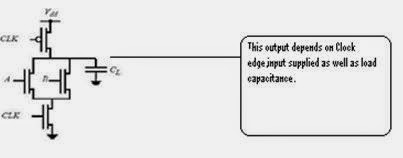## Thursday, July 24, 2014

### Static Vs Dynamic Logic Circuits

Comparison Between Dynamic Logic Circuit & Static Logic Circuit

• Dynamic logic circuit requires small area in comparison of static logic circuits.

• Dynamic logic circuit generates smaller parasitic capacitance, due to the smaller area on
silicon chip.

• These dynamic circuits has smaller dynamic power than static design because the less
parasitic capacitance.

• The common clock system in dynamic circuits in all over the circuits enables the
synchronization.

1) Static logic Gates: The output of these logic gates depends on its applied input levels.

The output can be keep as long as the power is supplied to circuit.

2) Dynamic Logic Gates: The output operation of these gates depends on the temporary

storage of the charge in the load capacitance. This stored charge does not stay for

indefinitely, it updates with the different voltage levels.

(Research Associate at Silicon Mentor)Dynamic Logic CircuitStatic Logic Circuit